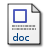# PENDEKATAN REGRESI UNTUK ANALISIS RAGAM KLASIFIKASI DUA-ARAH

Ispriyanti, Dwi (2007) PENDEKATAN REGRESI UNTUK ANALISIS RAGAM KLASIFIKASI DUA-ARAH. Jurnal Matematika dan Komputer, 10 (1). pp. 15-21. ISSN 1410-8518Microsoft Word - Published Version458Kb

## Abstract

Regression approach can be used for solving analysis of variance problems, wether of one way or two ways ANOVA . In ANOVA, model is an important factor. In this paper, we will study two ways classification ANOVA with regression approach for solving the fixed effect model. It can be done if the model is indentified truly and if the preventive procedure have been done so we have an independent normal equation. A characteristic of ANOVA is that the analysis model is overparameterized, so we have to make constraint for the parameters. In the Regression model approach for ANOVA problems, the independent variable X at categorical form 0 and 1 , or we have to make dummy variables at row and colom factors.

Item Type: Article ANOVA, constraint Q Science > QA Mathematics Faculty of Science and Mathematics > Department of Statistics 1835 Admin Departemen Matematika 30 Nov 2009 11:59 30 Nov 2009 11:59

Repository Staff Only: item control page